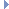﻿ MDynSweptTriangle Class Reference

# MDynSweptTriangle Class Reference

MDynSweptTriangle Class Reference

`#include <MDynSweptTriangle.h>`

## Class Description

Class for evaluating surfaces as triangles over time.

MDynSweptTriangle provides access to surfaces (Nurbs and Polys) defined as triangles. It can only be accessed with the MFnDynSweptGeometryData class that is provided as an output from the geoConnector node.

The class provides parametric time evaluation for a triangle. Time is in the range 0 to 1, where 1 represents the current frame and 0 represents the previous frame. In this way you can get interpolated values of a triangle in motion.Examples:

## Public Member Functions

MDynSweptTriangle ()
The class constructor.

~MDynSweptTriangle ()
The class destructor.

MVector vertex (int vertexId, double t=1.0)
Return the vertex requested by id, at the parametric time value. More...

MVector normal (double t=1.0)
Given a parametric time specified by 't', returns the normal of the triangle. More...

MVector normalToPoint (double x, double y, double z, double t=1.0)
Given a point, returns the normal of the triangle in the direction towards the point. More...

MVector uvPoint (int vertexId)
Given a vertex id, this method returns the UV point for the vertex as a MVector. More...

double area ()
This method returns the area. More...

## Static Public Member Functions

static const char * className ()
Returns the name of this class. More...

## Member Function Documentation

 MVector vertex ( int vertexId, double t = `1.0` )

Return the vertex requested by id, at the parametric time value.

Parameters
 [in] vertexId index 0, 1, or 2 [in] t time value in the range 1.0 to 0.0 t=1.0 is the current frame t=0.0 is the previous frame
Returns
The vertex position.Examples:
 MVector normal ( double t = `1.0` )

Given a parametric time specified by 't', returns the normal of the triangle.

Parameters
 [in] t time value in the range 1.0 to 0.0 t=1.0 is the current frame t=0.0 is the previous frame
Returns
A normalized vector
 MVector normalToPoint ( double x, double y, double z, double t = `1.0` )

Given a point, returns the normal of the triangle in the direction towards the point.

Determines which side of the triangle to return the normal.

Parameters
 [in] x x component of the point [in] y y component of the point [in] z z component of the point [in] t time value in the range 1.0 to 0.0 t=1.0 is the current frame t=0.0 is the previous frame
Returns
A normalized vector
 MVector uvPoint ( int vertexId )

Given a vertex id, this method returns the UV point for the vertex as a MVector.

Parameters
 [in] vertexId Id of the vertex for retrieving the UV point.
Returns
A vector. The u and v values are stored in the first two coordinates. The third coordinate is not meaningful.Examples:
 double area ( )

This method returns the area.

Returns
The area, as double value.Examples:
 const char * className ( )
static

Returns the name of this class.

Returns
The name of this class.

The documentation for this class was generated from the following files:
• MDynSweptTriangle.h
• MDynSweptTriangle.cpp